This function can fit a time series model to data using different methods.

estimate(model, Xt, method = "mle", demean = TRUE)

## Arguments

model

A time series model.

Xt

A vector of time series data.

method

A string indicating the method used for model fitting. Supported methods include mle, yule-walker, gmwm and rgmwm.

demean

A boolean indicating whether the model includes a mean / intercept term or not.

## Author

Stéphane Guerrier and Yuming Zhang

## Examples

Xt = gen_gts(300, AR(phi = c(0, 0, 0.8), sigma2 = 1))
plot(Xt)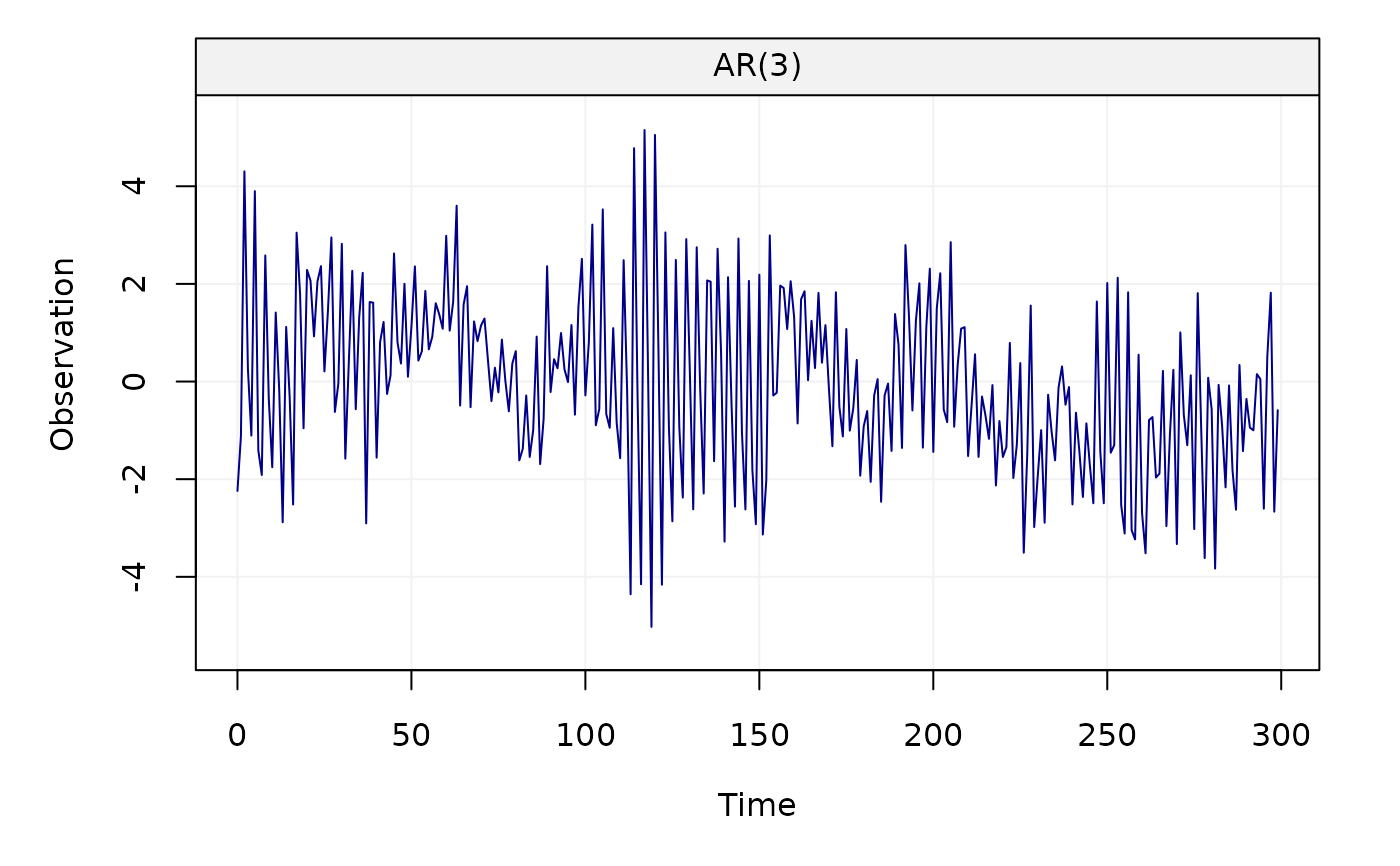estimate(AR(3), Xt)
#> Fitted model: AR(3)
#>
#> Estimated parameters:
#>
#> Call:
#> arima(x = as.numeric(Xt), order = c(p, intergrated, q), seasonal = list(order = c(P,
#>     seasonal_intergrated, Q), period = s), include.mean = demean, method = meth)
#>
#> Coefficients:
#>           ar1      ar2     ar3  intercept
#>       -0.0274  -0.0272  0.8255    -0.0507
#> s.e.   0.0327   0.0327  0.0326     0.2510
#>
#> sigma^2 estimated as 1.061:  log likelihood = -436.26,  aic = 882.53

Xt = gen_gts(300, MA(theta = 0.5, sigma2 = 1))
plot(Xt)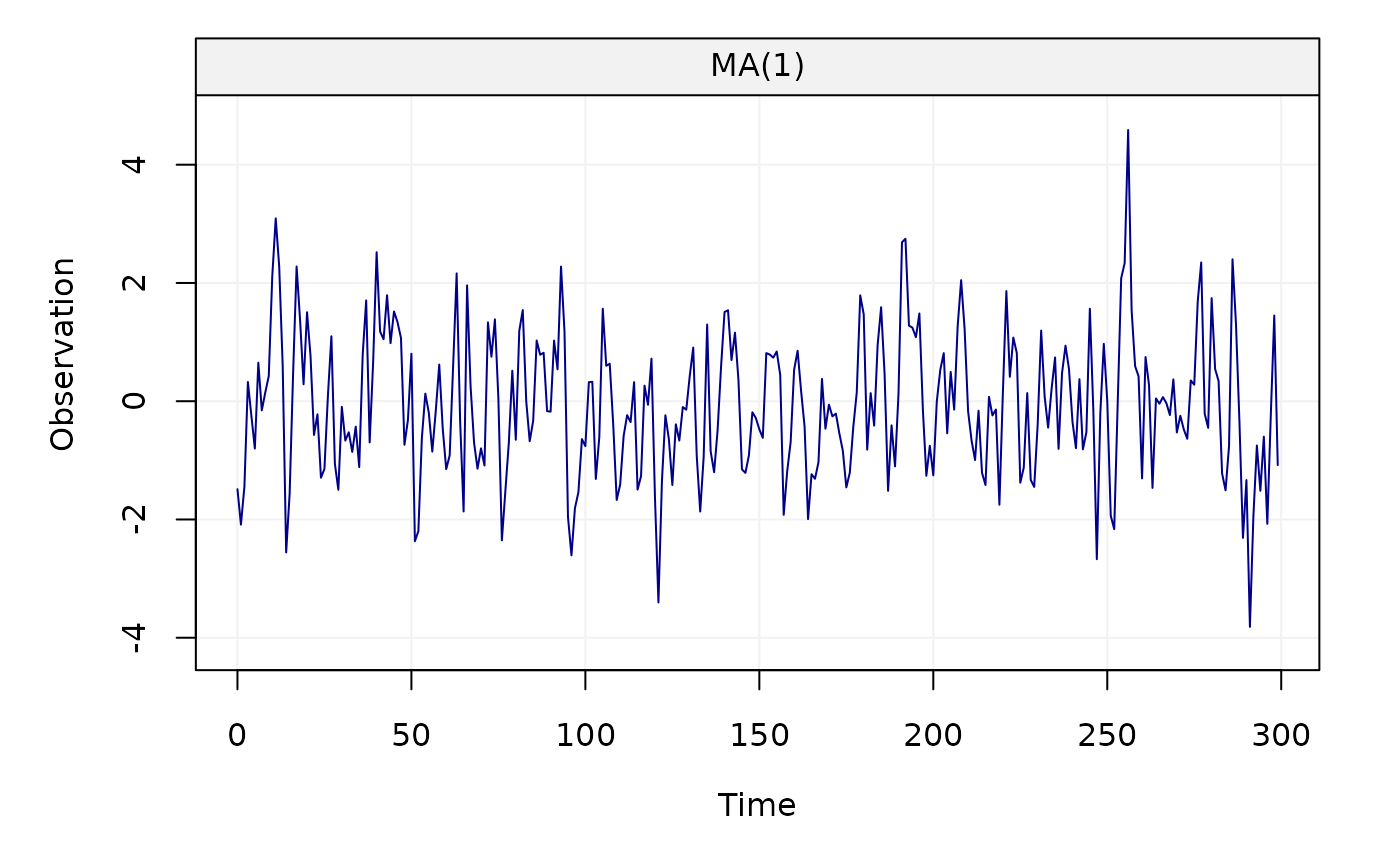estimate(MA(1), Xt, method = "gmwm")
#> Fitted model: MA(1)
#>
#> Estimated parameters:
#> Model Information:
#>        Estimates
#> MA     0.6735315
#> SIGMA2 0.9822984
#>
#> * The initial values of the parameters used in the minimization of the GMWM objective function
#>   were generated by the program underneath seed: 1337.
#>

Xt = gen_gts(300, ARMA(ar = c(0.8, -0.5), ma = 0.5, sigma2 = 1))
plot(Xt)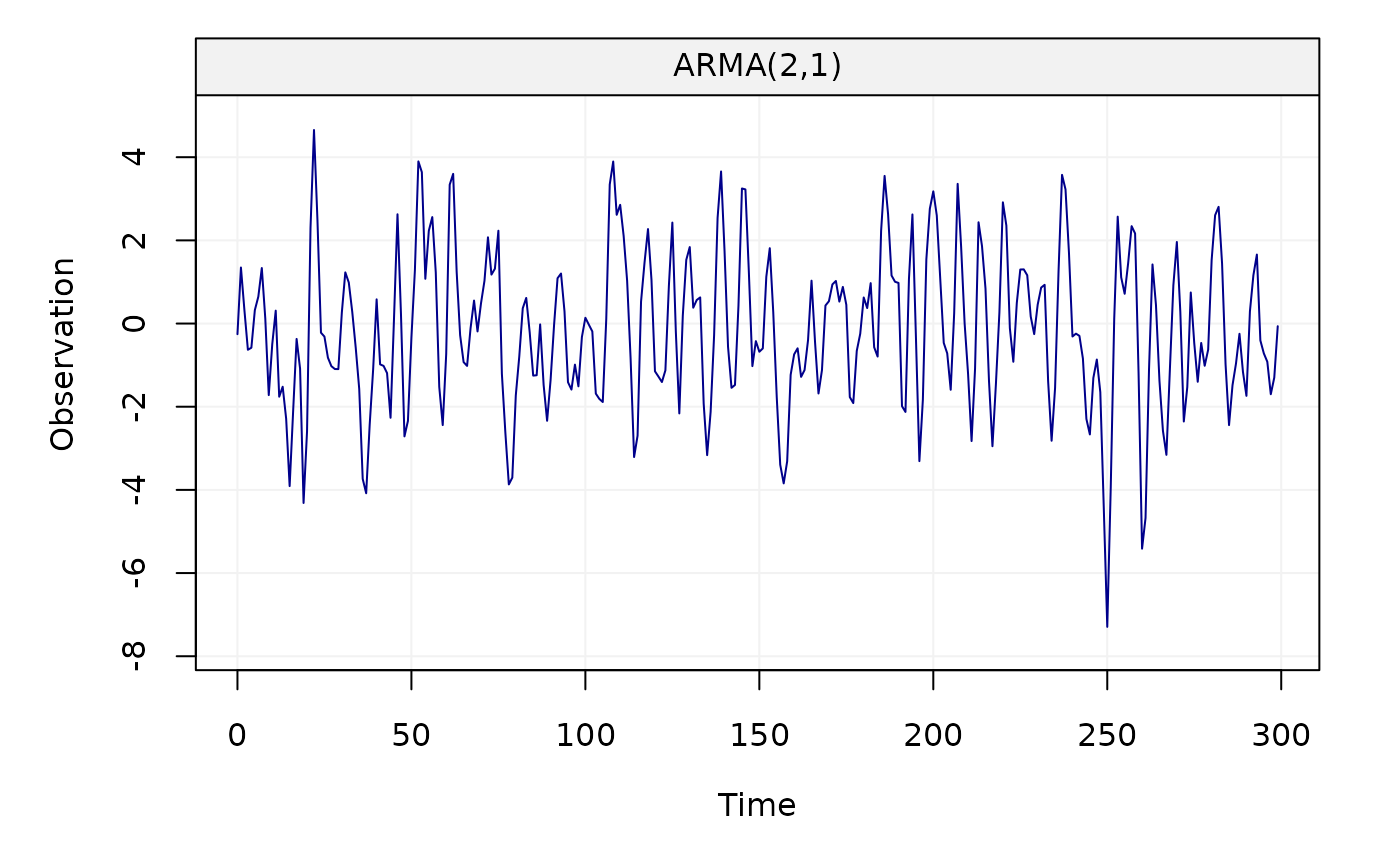estimate(ARMA(2,1), Xt, method = "rgmwm")
#> Fitted model: ARMA(2,1)
#>
#> Estimated parameters:
#> Model Information:
#>         Estimates
#> AR      0.7640776
#> AR     -0.4382348
#> MA      0.5143711
#> SIGMA2  1.1560304
#>
#> * The initial values of the parameters used in the minimization of the GMWM objective function
#>   were generated by the program underneath seed: 1337.
#>

Xt = gen_gts(300, ARIMA(ar = c(0.8, -0.5), i = 1, ma = 0.5, sigma2 = 1))
plot(Xt)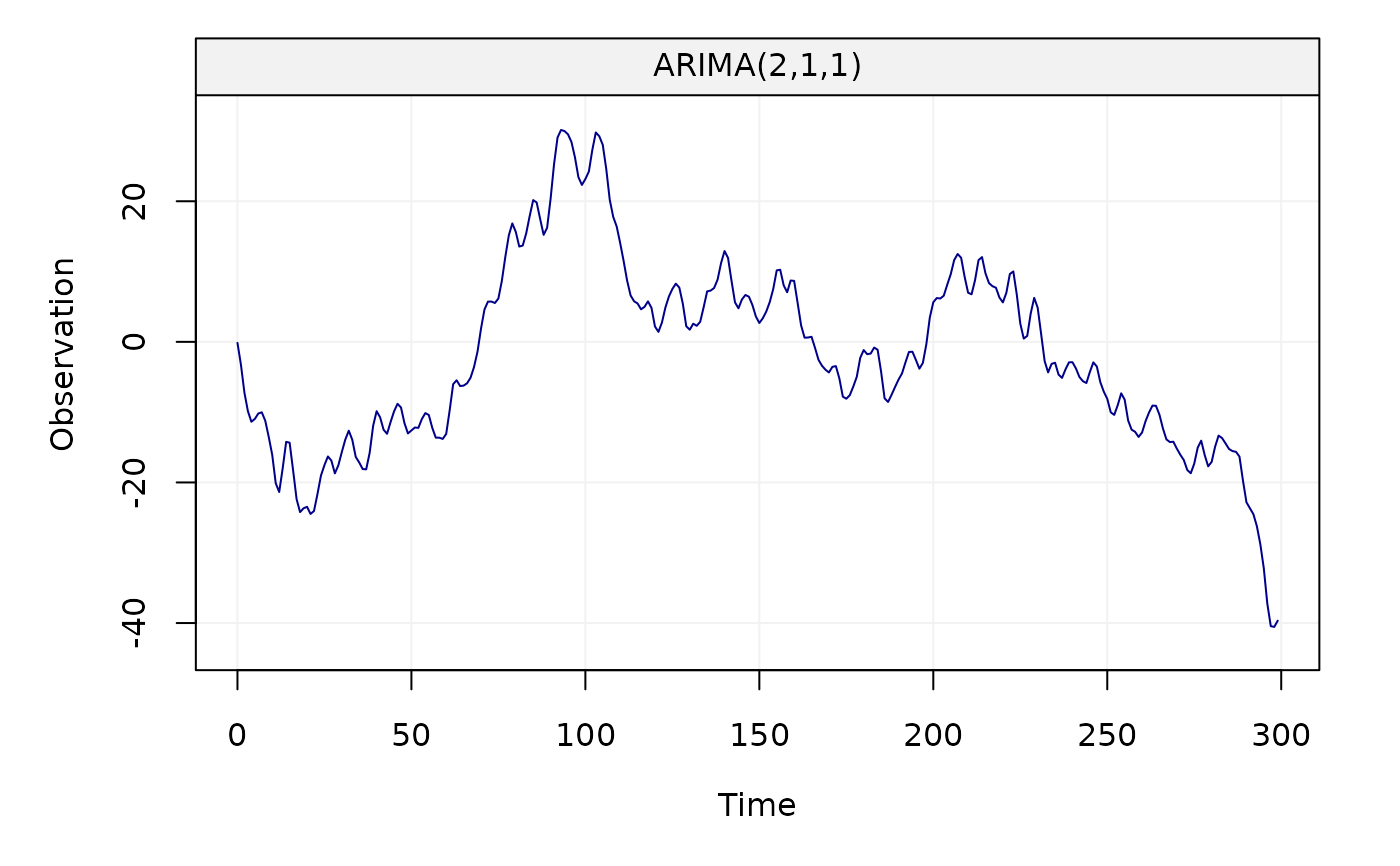estimate(ARIMA(2,1,1), Xt, method = "mle")
#> Fitted model: ARIMA(2,1,1)
#>
#> Estimated parameters:
#>
#> Call:
#> arima(x = as.numeric(Xt), order = c(p, intergrated, q), seasonal = list(order = c(P,
#>     seasonal_intergrated, Q), period = s), include.mean = demean, method = meth)
#>
#> Coefficients:
#>          ar1      ar2     ma1
#>       0.8049  -0.4668  0.5264
#> s.e.  0.0649   0.0613  0.0619
#>
#> sigma^2 estimated as 0.9961:  log likelihood = -424.71,  aic = 857.42

Xt = gen_gts(1000, SARIMA(ar = c(0.5, -0.25), i = 0, ma = 0.5, sar = -0.8,
si = 1, sma = 0.25, s = 24, sigma2 = 1))
plot(Xt)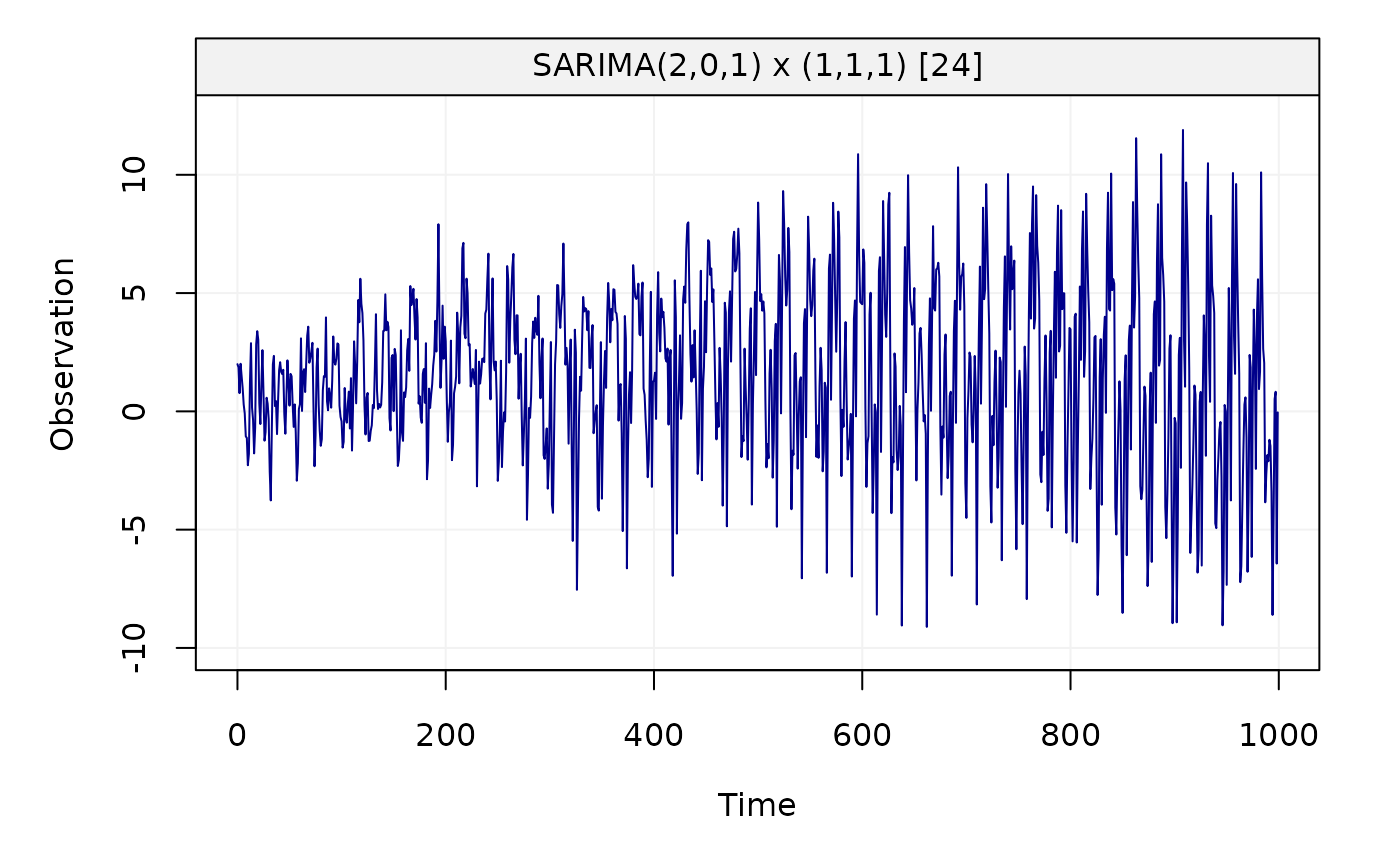estimate(SARIMA(ar = 2, i = 0, ma = 1, sar = 1, si = 1, sma = 1, s = 24), Xt,
method = "rgmwm")
#> Fitted model: SARIMA(2,0,1) x (1,1,1) 
#>
#> Estimated parameters:
#> Model Information:
#>         Estimates
#> AR      0.3723398
#> AR     -0.2431420
#> MA      0.5590085
#> SAR    -0.7706956
#> SMA     0.1365543
#> SIGMA2  0.7827080
#>
#> * The initial values of the parameters used in the minimization of the GMWM objective function
#>   were generated by the program underneath seed: 1337.
#>